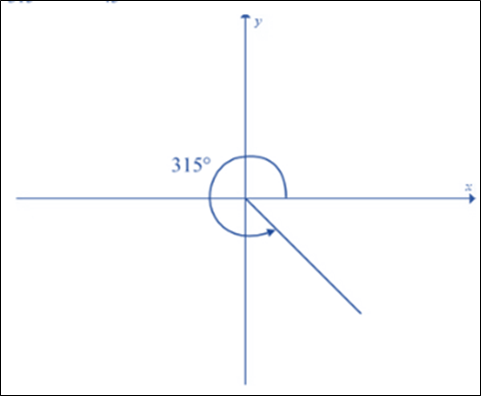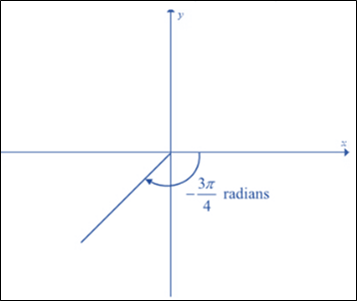# To graph: The given angle in the standard position: 315 °### Single Variable Calculus: Concepts...

4th Edition
James Stewart
Publisher: Cengage Learning
ISBN: 9781337687805### Single Variable Calculus: Concepts...

4th Edition
James Stewart
Publisher: Cengage Learning
ISBN: 9781337687805

#### Solutions

Chapter C, Problem 9E

(a)

To determine

## To graph: The given angle in the standard position:   315°

Expert Solution

### Explanation of Solution

Given information:

315°

Graph:Interpretation:

The given value of radian is 315° . It is positive angle value that means the rotation is measured from the x axis as counter-clockwise direction.

Know that:

In one full rotation the angle is 360° .

Now,

315°=36045

Thus, the given angle 315° measures rotation of full revolution less than 45° from the positive x axis.

(b)

To determine

Expert Solution

### Explanation of Solution

Given information:

Graph:Interpretation:

The given value of radian is 3π4rad . It is negative angle value that means the rotation is measured from the x axis as clockwise direction.

Know that:

In one full rotation the angle is 2π . And the relation between the degree and radian is shown by below expression: